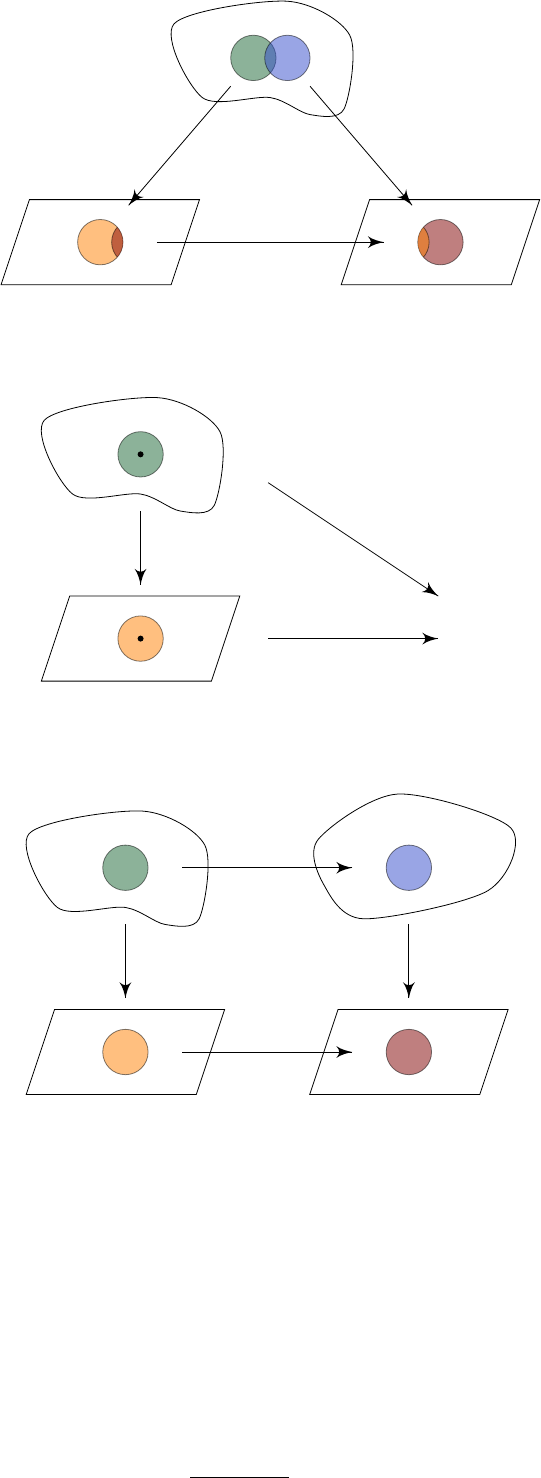2Lie groups

III Symmetries, Fields and Particles2.1 Definitions
A Lie group is a group and a manifold, where the group operations define smooth
maps. We already know what a group is, so let’s go into a bit more details about
what a manifold is.
The canonical example of a manifold one might want to keep in mind is the
sphere
S
2
. Having lived on Earth, we all know that near any point on Earth,
S
2
looks like
R
2
. But we also know that
S
2
is not
R
2
. We cannot specify a point
on the surface of the Earth uniquely by two numbers. Longitude/latitude might
be a good start, but adding 2
π
to the longitude would give you the same point,
and things also break down at the poles.
Of course, this will not stop us from producing a map of Cambridge. One
can reasonably come up with consistent coordinates just for Cambridge itself.
S
2
is that near each point, we can come up with some
coordinate system, but we cannot do so for the whole space itself.
Definition
(Smooth map)
.
We say a map
f
:
R
n
R
m
is smooth if all partial
derivatives of all orders exist.
Definition
(Manifold)
.
A manifold (of dimension
n
) is a set
M
together with
the following data:
(i) A collection U
α
of subsets of M whose union is M;
(ii)
A collection of bijections
ϕ
α
:
U
α
V
α
, where
V
α
is an open subset of
R
n
.
These are known as charts.
The charts have to satisfy the following compatibility condition:
For all
α, β
, we have
ϕ
α
(
U
α
U
β
) is open in
R
n
, and the transition function
ϕ
α
ϕ
1
β
: ϕ
β
(U
α
U
β
) ϕ
α
(U
α
U
β
)
is smooth.
We write (M, ϕ
α
) for the manifold if we need to specify the charts explicitly.
U
β
U
α
ϕ
β
ϕ
α
ϕ
α
ϕ
1
β
Definition
(Smooth map)
.
Let
M
be a manifold. Then a map
f
:
M R
is smooth if it is smooth in each coordinate chart. Explicitly, for each chart
(U
α
, ϕ
α
), the composition f ϕ
1
α
: ϕ
α
(U
α
) R is smooth (in the usual sense)
p
U
ϕ(p)
f ϕ
1
R
f
ϕ
More generally, if
M, N
are manifolds, we say
f
:
M N
is smooth if for
any chart (
U, ϕ
) of
M
and any chart (
V, ξ
) of
N
, the composition
ξ f ϕ
1
:
ϕ(U) ξ(V ) is smooth.
ϕ
ξ
f
ξ f ϕ
1
Finally, we note that if
M, N
are manifolds of dimensions
m
and
n
respectively,
then
M × N
is a manifold of dimension
m
+
n
, where charts (
U, ϕ
:
U
R
m
)
,
(
V, ξ
:
V R
n
) of
M
and
N
respectively give us a chart
ϕ × ξ
:
U × V
R
m
× R
n
= R
m+n
of M × N .
All those definitions were made so that we can define what a Lie group is:
Definition
(Lie group)
.
A Lie group is a group
G
whose underlying set is given
a manifold structure, and such that the multiplication map
m
:
G ×G G
and
inverse map
i
:
G G
are smooth maps. We sometimes write
M
(
G
) for the
underlying manifold of G.
Example. The unit 2-sphere
S
2
= {(x, y, z) R
3
: x
2
+ y
2
+ z
2
= 1}
is a manifold. Indeed, we can construct a coordinate patch near
N
= (0
,
0
,
1).
Near this point, we have
z
=
p
1 x
2
y
2
. This works, since near the north pole,
the
z
-coordinate is always positive. In this case,
x
and
y
are good coordinates
near the north pole.
However, it is a fact that the 2-sphere S
2
has no Lie group structure.
In general, most of our manifolds will be given by subsets of Euclidean space
specified by certain equations, but note that not all subsets given by equations
are manifolds! It is possible that they have some singularities.
Definition
(Dimension of Lie group)
.
The dimension of a Lie group
G
is the
dimension of the underlying manifold.
Definition
(Subgroup)
.
A subgroup
H
of
G
is a subset of
G
that is also a group
under the same operations. We write H G if H is a subgroup of G.
The really interesting thing is when the subgroup is also a manifold!
Definition
(Lie subgroup)
.
A subgroup is a Lie subgroup if it is also a manifold
(under the induced smooth structure).
We now look at some examples.
Example.
Let
G
= (
R
D
,
+) be the
D
as the group operation. The inverse of a vector
x
is
x
, and the identity is
0
.
This is obviously locally homeomorphic to
R
D
, since it is
R
D